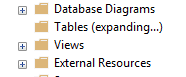# SQL Server / SSMS / Expanding Tables List Query

Usually I do not even notice this query working in the background, since this operation is quite fast. But recently I end up working with a customer having DB with 150K tables, and expanding table list operation can be really slow and expensive.Here is the code:

```SELECT
tbl.name AS [Name],
SCHEMA_NAME(tbl.schema_id) AS [Schema],
'Server[@Name=' + quotename(CAST(
serverproperty(N'Servername')
AS sysname),'''') + ']' + '/Database[@Name=' + quotename(db_name(),'''') + ']' + '/Table[@Name=' + quotename(tbl.name,'''') + ' and @Schema=' + quotename(SCHEMA_NAME(tbl.schema_id),'''') + ']' AS [Urn],
tbl.create_date AS [CreateDate],
CAST(tbl.is_memory_optimized AS bit) AS [IsMemoryOptimized],
CAST(CASE idx.type WHEN 5 THEN 1 ELSE 0 END AS bit) AS [HasClusteredColumnStoreIndex],
CAST(tbl.is_remote_data_archive_enabled AS bit) AS [RemoteDataArchiveEnabled],
tbl.temporal_type AS [TemporalType],
CAST(CASE WHEN 'PS'=dsidx.type THEN 1 ELSE 0 END AS bit) AS [IsPartitioned],
CAST(
ISNULL((SELECT distinct 1 from sys.all_columns
WHERE object_id = tbl.object_id
AND is_sparse = 1), 0)
AS bit) AS [HasSparseColumn],
CAST(tbl.is_node AS bit) AS [IsNode],
CAST(tbl.is_edge AS bit) AS [IsEdge],
CAST(ISNULL((select top 1 1 from sys.indexes ind where ind.object_id = tbl.object_id and ind.type > 1 and ind.is_hypothetical = 0 ), 0) AS bit) AS [HasNonClusteredIndex],
CAST(CASE idx.index_id WHEN 1 THEN 1 ELSE 0 END AS bit) AS [HasClusteredIndex],
CAST(case idx.index_id when 1 then case when (idx.is_primary_key + 2*idx.is_unique_constraint = 1) then 1 else 0 end else 0 end AS bit) AS [HasPrimaryClusteredIndex],
CAST(ISNULL((select top 1 1 from sys.indexes ind where ind.object_id = tbl.object_id and ind.type = 3 and ind.is_hypothetical = 0 ), 0) AS bit) AS [HasXmlIndex],
CAST(ISNULL((select top 1 1 from sys.all_columns as clmns join sys.types as usrt on usrt.user_type_id = clmns.user_type_id where clmns.object_id = tbl.object_id and usrt.name = N'xml'), 0) AS bit) AS [HasXmlData],
CAST(ISNULL((select top 1 1 from sys.all_columns as clmns join sys.types as usrt on usrt.user_type_id = clmns.user_type_id where clmns.object_id = tbl.object_id and usrt.name in (N'geometry', N'geography')), 0) AS bit) AS [HasSpatialData],
CAST(ISNULL((select top 1 1 from sys.indexes ind where ind.object_id = tbl.object_id and ind.type = 6 and ind.is_hypothetical = 0 ), 0) AS bit) AS [HasNonClusteredColumnStoreIndex]
FROM
sys.tables AS tbl
INNER JOIN sys.indexes AS idx ON
idx.object_id = tbl.object_id and (idx.index_id < @_msparam_0  or (tbl.is_memory_optimized = 1 and idx.index_id = (select min(index_id) from sys.indexes where object_id = tbl.object_id)))```

Query plan: